### Creating and Decorating Scatterplot in ggplot2 in RHello and welcome to another interesting article. This article emphasizes on creating a Scatterplot and decorating it using different options supplied by ggplot2 system. Here is the overview of this article:

## Data

To demonstrate how to create a scatterplot, we will use the data that we have prepared in our previous post "How to prepare data for analysis in R in 5 steps". As well as, all examples below will only use data frames. Original data is available to download from s and p 500 companies financials. This data is available under the PDDL license.

Let’s first create our data set by reading the file using `read.csv` function and check the name of the variables using `names` function.

``````financials <- read.csv("constituents-financials_csv.csv")
names(financials)``````
``````##   "Symbol"         "Name"           "Sector"         "Price"
##   "Price.Earnings" "Dividend.Yield" "Earnings.Share" "X52.Week.Low"
##   "X52.Week.High"  "Market.Cap"     "EBITDA"         "Price.Sales"
##  "Price.Book"     "SEC.Filings"``````

Now when we are familiar with the data set, let’s first create the empty ggplot object and start layering it.

## Creating a ggplot object

The code below creates an empty plot using `financial` data frame. To add information to the plot, we will have to start adding aesthetics. The plot we are making will display a correlation between price and dividend.

``````pt <- ggplot(financials)
plot(pt)``````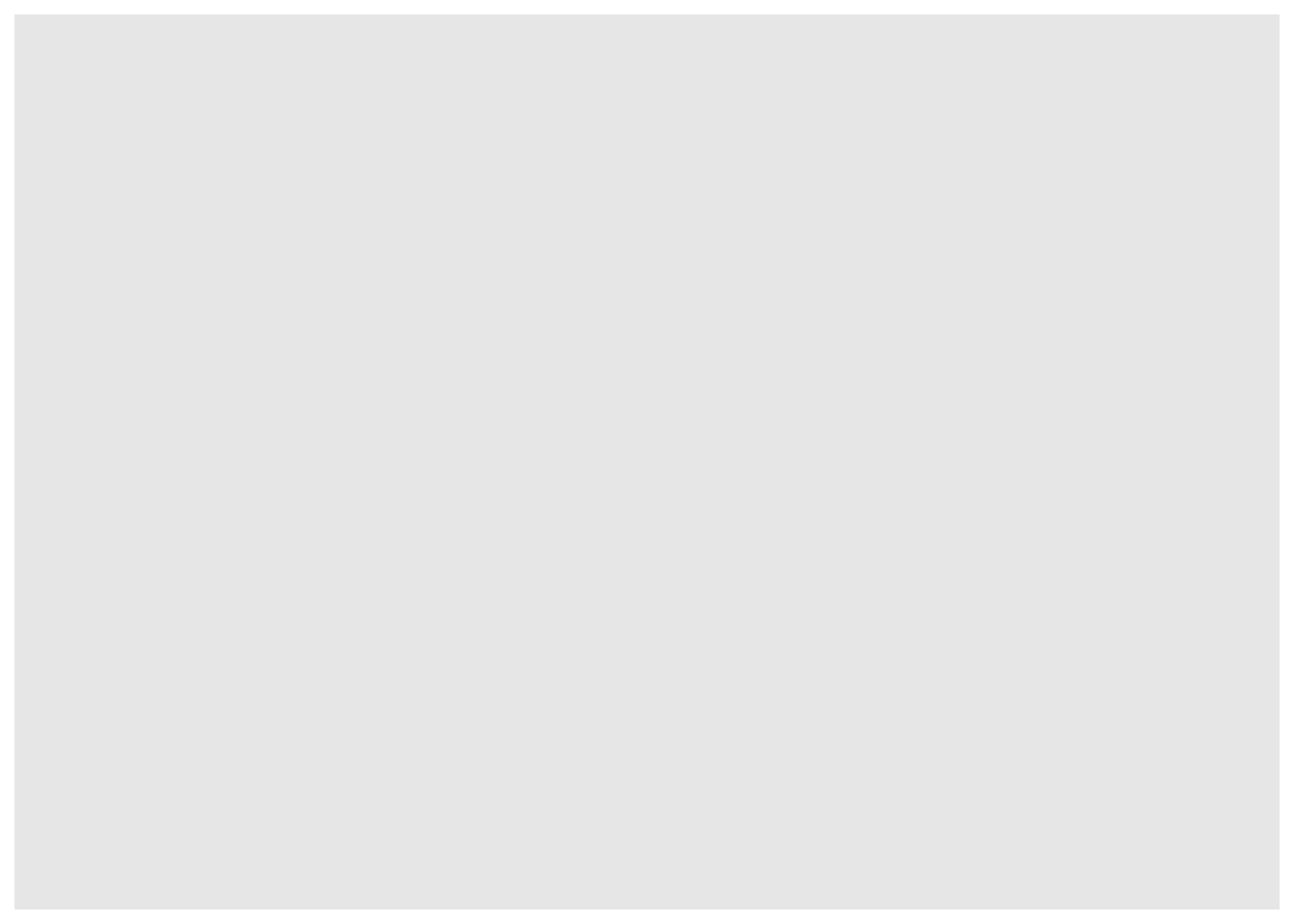Aesthetics as an adjective means “concerned with beauty or the appreciation of beauty”. Aesthetics are the properties of the plot. These properties could be related to the axis and other decorative elements like colour, title, subtitle, caption etc. Let’s assign variables price to x-axis and dividend to the y-axis and show points of intersection by variable sector in different colours. Note that the legend you would see on the plot is auto-generated and automatically shows unique values.

Aesthetics are always part of a geom which is a geometric object which ggplot system use to plot the data. The use of specific geom depends on the type of presentation is required. Here we are plotting a Scatterplot and hence we have chosen `geom_point` to show intersections of x and y-axis in points or dots.

``````pt <- ggplot(financials, aes(x=Price, y=Dividend.Yield)) +
geom_point(aes(col=Sector))
plot(pt)``````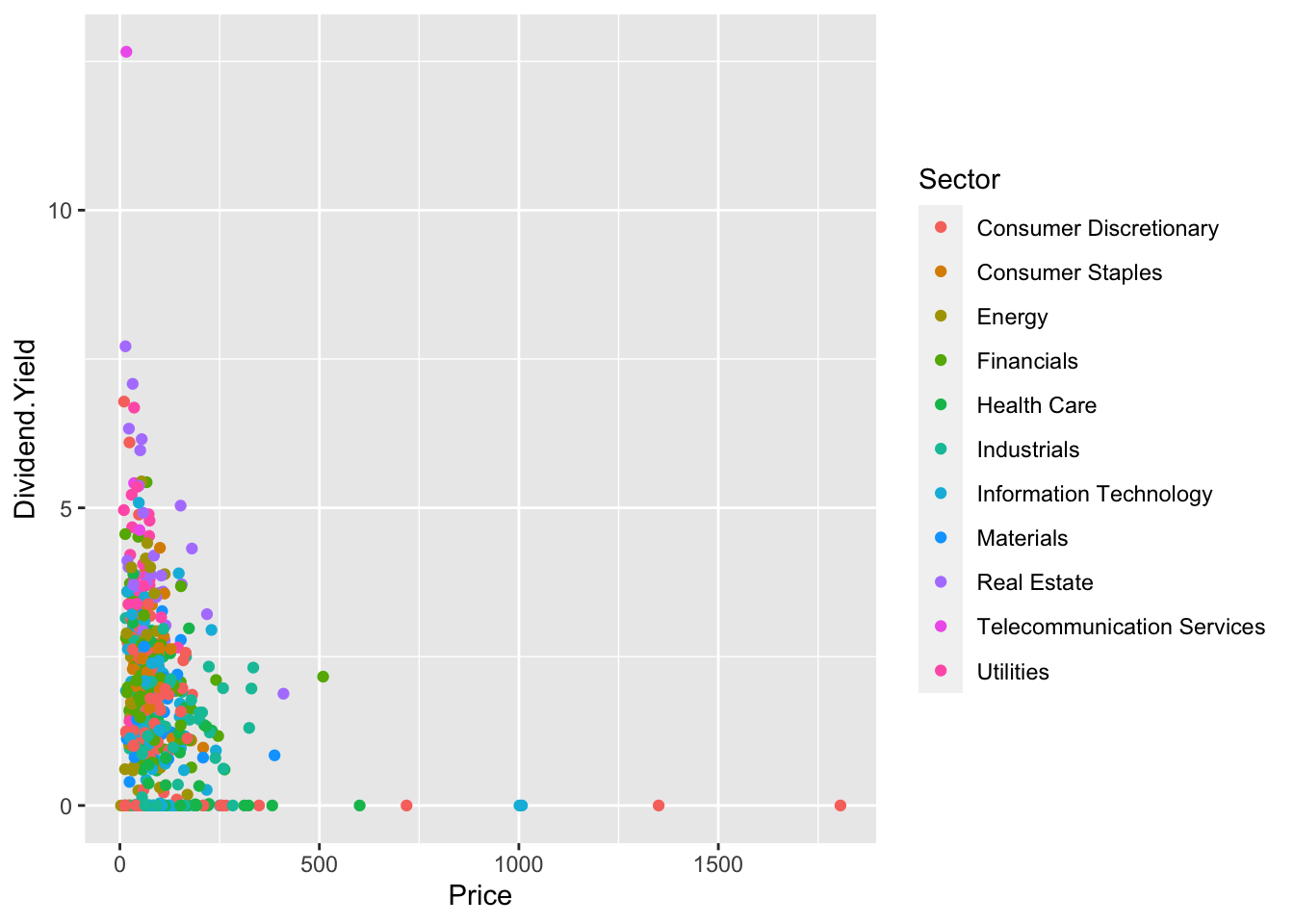In addition, your plot is not limited to have just one geom, you can add geom based on your requirements and one good example is to have a regression or trend line. A regression or trend line can be added using geom_smooth function. By default, `geom_smooth` shows a smooth with confidence band that is visible around the trend line. This confidence band can be switched off using `se=FALSE` clause. We can define the colour and type of line as well. Let’s have a look.

``````pt <- ggplot(financials, aes(x=Price, y=Dividend.Yield)) +
geom_point(aes(col=Sector)) +
geom_smooth(se=FALSE, color="red", linetype="solid")
plot(pt)``````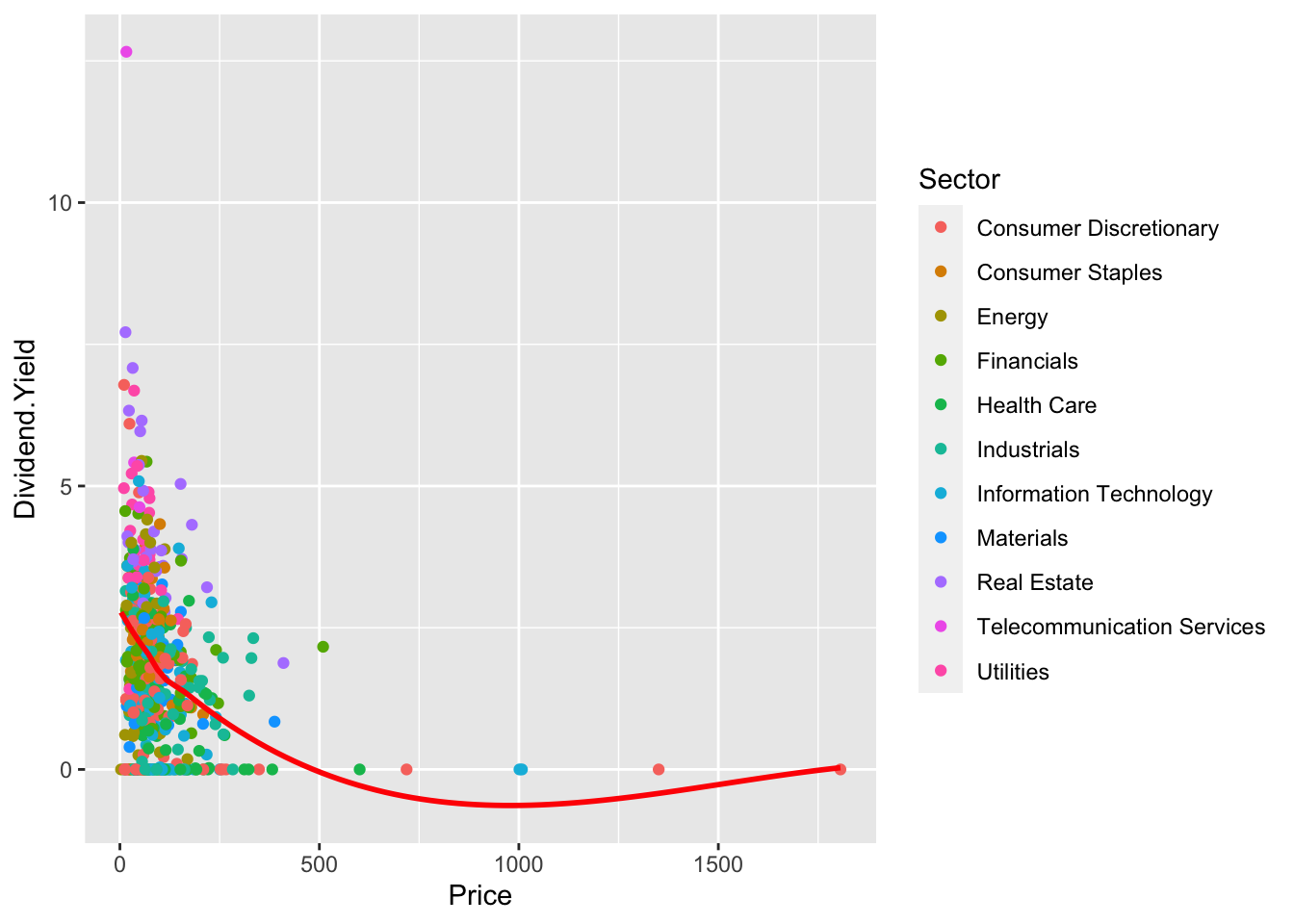## Removing legends from Scatterplot

By default, legends appear when you plot Scatterplots and ggplot2 plot systems show unique values from the chosen variable. However, if you want to switch off the legends then you can do it by setting show.legend clause to FALSE.

``````pt <- ggplot(financials, aes(x=Price, y=Dividend.Yield)) +
geom_point(aes(col=Sector), show.legend=FALSE) +
geom_smooth(se=FALSE)
plot(pt)``````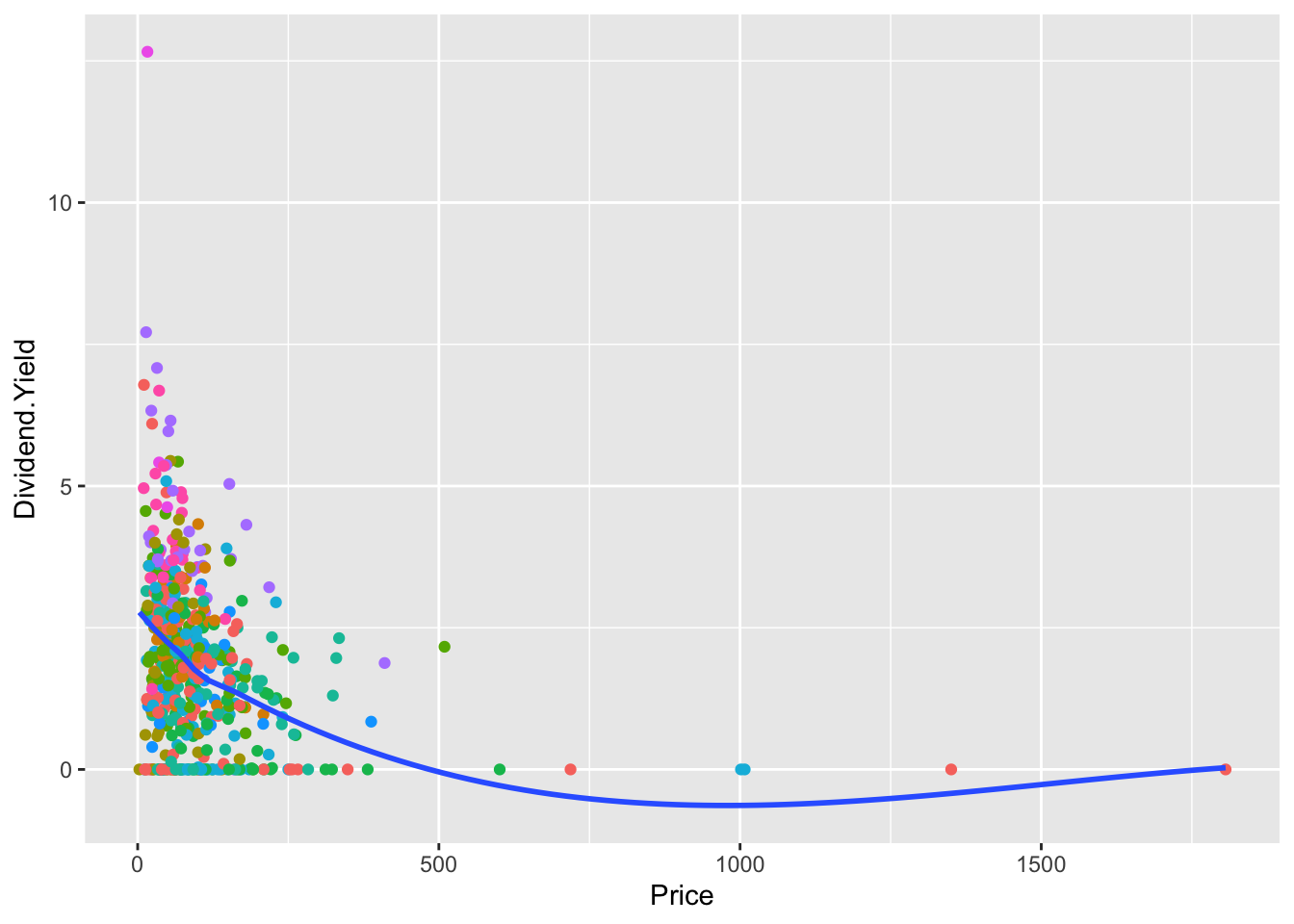## Adding title, subtitle and caption to Scatterplot

Title of a plot is important and it gives the audience a context about the information being presented. Furthermore, `title``subtitle` and `caption` can all be added to the scatterplot using `labs` function. Additionally, `theme` function can be used to define the different properties of these labels. Have a look at the example below showing alignment, colour, size and font face properties.

``````pt <- ggplot(financials, aes(x=Price, y=Dividend.Yield)) +
geom_point(aes(col=Sector), show.legend=FALSE) +
geom_smooth(se=FALSE) +
labs(title="Scatterplot Example",
subtitle="S and P 500 companies financials. Price v/s Dividend Yield",
theme(plot.title = element_text(hjust = 0, color="blue", size=14, face="bold"),
plot.subtitle = element_text(hjust = 0, color="black", size=12, face="italic"),
plot.caption = element_text(hjust = 0.5, color="blue", size=10, face="plain"))
plot(pt)``````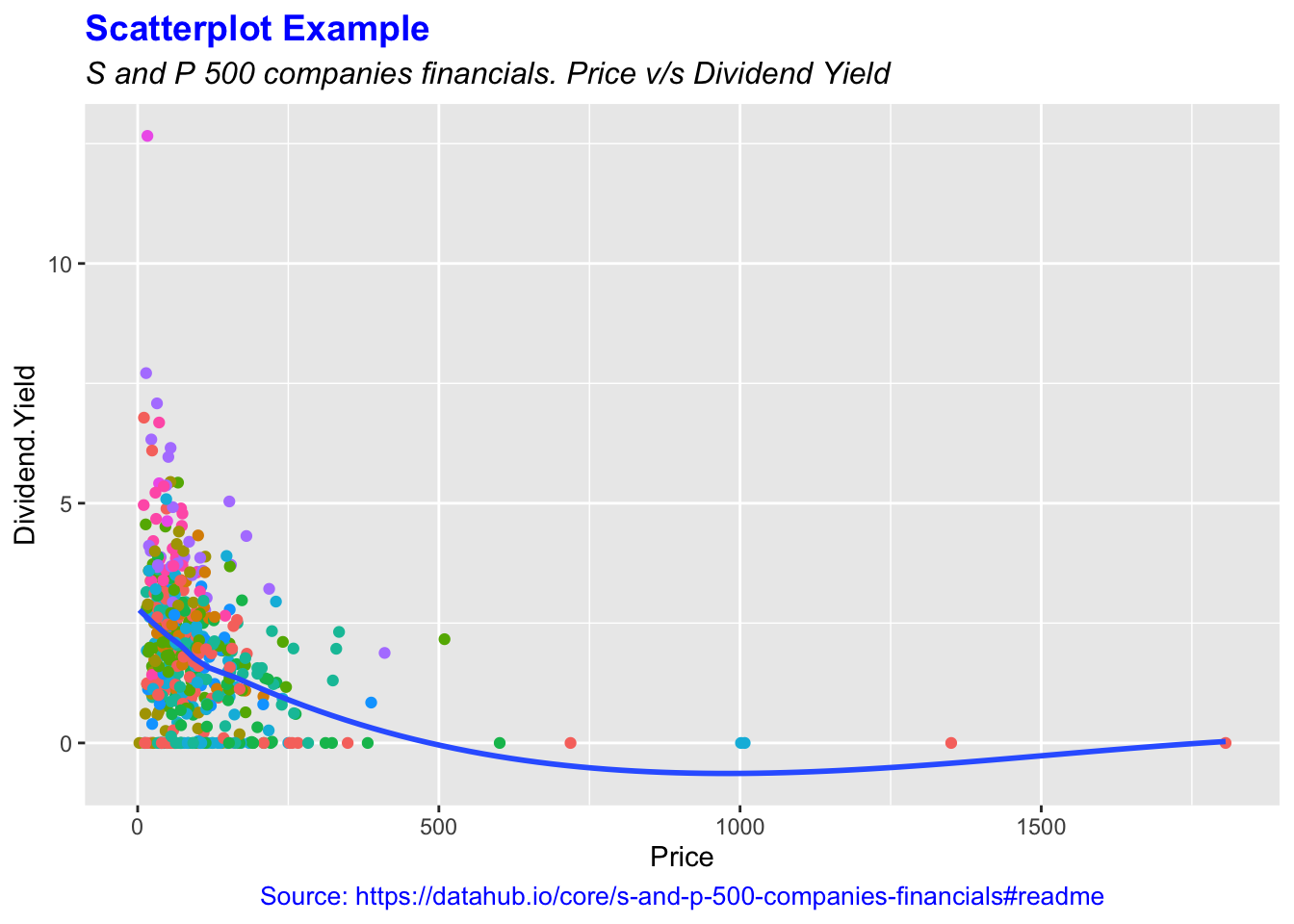Note in the example above, clause `hjust` is used for alignment. 0 is used to define left, 1 is for right and 0.5 for the centre. Furthermore, labels for x and y-axis have not to be defined explicitly but if you want to change them then they can be added inside labs function.

``````pt <- ggplot(financials, aes(x=Price, y=Dividend.Yield)) +
geom_point(aes(col=Sector), show.legend=FALSE) +
geom_smooth(se=FALSE) +
labs(title="Scatterplot Example",
subtitle="S and P 500 companies financials. Price v/s Dividend Yield",
x = "Price",
y = "Dividend.Yield") +
theme(plot.title = element_text(hjust = 0, color="blue", size=14, face="bold"),
plot.subtitle = element_text(hjust = 0, color="black", size=12, face="italic"),
plot.caption = element_text(hjust = 0.5, color="blue", size=10, face="plain"))
plot(pt)``````## Printing plot on a device

Now when we have the plot ready, we can print it on multiple devices. These devices can be like screen, PDF file device, image file device or vector graphics file device. Here in this section, we will print the plot as a PNG file device. By default, R uses the screen device and to print the plot to PNG device we will open the PNG device just before the plot function call.

``````pt <- ggplot(financials, aes(x=Price, y=Dividend.Yield)) +
geom_point(aes(col=Sector), show.legend=FALSE) +
geom_smooth(se=FALSE) +
labs(title="Scatterplot Example",
subtitle="S and P 500 companies financials. Price v/s Dividend Yield",
x = "Price",
y = "Dividend.Yield") +
theme(plot.title = element_text(hjust = 0, color="blue", size=14, face="bold"),
plot.subtitle = element_text(hjust = 0, color="black", size=12, face="italic"),
plot.caption = element_text(hjust = 0.5, color="blue", size=10, face="plain"))
# Define device, set characteristics and plot
png("Scatterplot.png", 480, 480)
plot(pt)
# set the device off and back to the default screen device
dev.off()``````
I hope you liked this post. If you do then please do comment and share it with your network. If you wish you can download the .R file containing the code explained above from dataENQ GitHub repository.

Here is the final plot.

View this post on Instagram

A post shared by dataenq (@dataenq) on